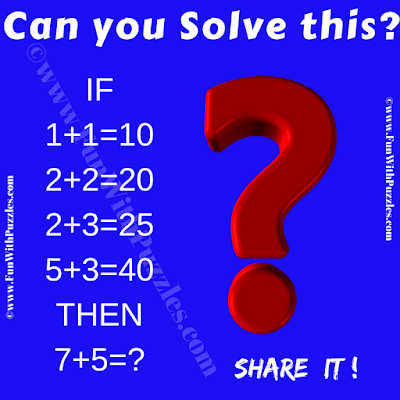This Math Logic Puzzle is for school-going Kids. This Math Logic Puzzle will test your brain. In this Puzzle, you are given some number equations. Your challenge is to decipher the hidden logical pattern in these equations and then find the value of the missing number which will replace the question mark. Can you take this challenge and solve this Brain Testing Math Logic Puzzle?Can you solve this Math Logic Puzzle?

The answer to this "Brain Testing Math Logic Puzzle", can be viewed by clicking on the answer button.